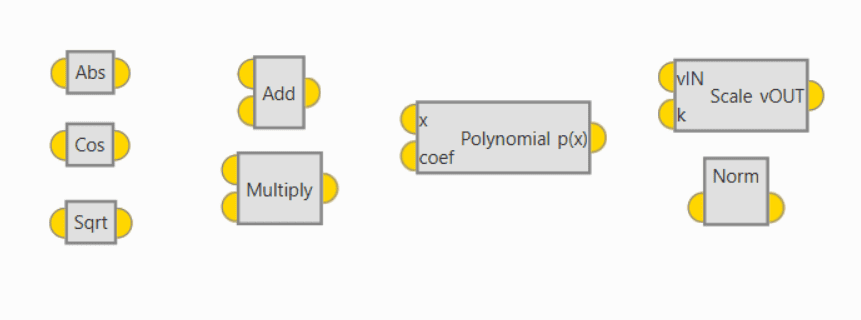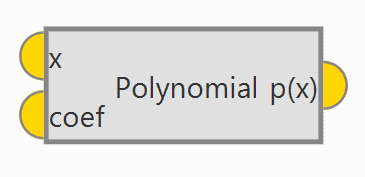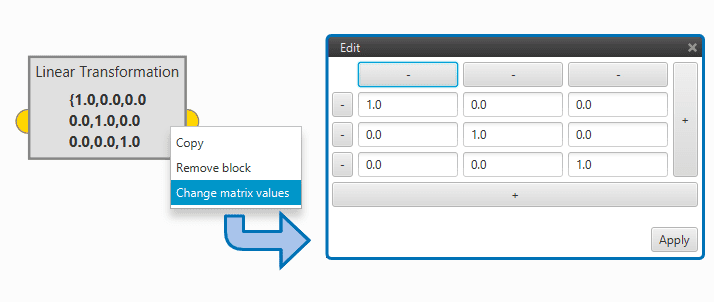# Math Blocks¶

Math blocks allow to perform a wide variety of mathematical operations.Math Blocks

1R –> 1R

1-input 1-output blocks

• Abs: returns absolute value of the input.

• Sin: returns the sine of an angle (in rad).

• Cos: returns the cosine of an angle (in rad).

• Tan: returns the tangent of an angle (in rad).

• Arcsin: inverse function of the sine. It returns an angle (in rad).

• Arccos: inverse function of the cosine. It returns an angle (in rad).

• Arctan: inverse function of the tangent. It returns an angle (in rad).

• Sqrt: returns the square root of the input.

• Exp: returns $$e$$ powered to the input.

• Log: returns the natural logarithm of the input.

• Ceil: returns the input, rounded up.

• Floor: returns the input, rounded down.

• [pi,-pi]Unwrap: return the unwrap of the input. That is, if a jump higher than $$\pi$$ is detected, an offset of $$(-)2\pi$$ is added to the result.

• [pi,-pi]Wrap: returns an angle (in rad) wrapped between $$\pi$$ and $$-\pi$$.

• [0,2pi]Wrap: returns an angle (in rad) wrapped between $$0$$ and $$2\pi$$.

2R –> 1R

2-input 1-output blocks

• Add: returns the sum of the inputs.

• Substract: returns the substraction of the inputs.

• Multiply: returns the product of the inputs.

• Divide: returns the division of the inputs.

• Power: returns input 1 to the power of input 2.

• Max: returns highest of the inputs.

• Min: returns the lowest of the inputs.

• Remainder: returns the remainder of the division of the inputs.

• Atan2: variation of the atan function, that allows to avoid .

Polynomial

Returns the value of the polinomial defined by the coefficients coef for the value of x.Polynomial Block

Vectors

Perform operations with vectors

• Add: return the sum of the input vectors.

• Add elements: returns the sum of input vector components.

• Bundle: returns a vector whose components are the inputs to the block.

• Dot product: returns the dot product of the input vectors.

• Linear transformation: returns the input vector multiplied by the transformation matrix. In order to edit the transformation matrix, double click on the block.Linear Transformation Block

• Multiply elements: returns the product of the vector input components.

• Norm: returns the norm of the input vector.

• Max: returns the value and position of the highest component of the input vector.

• Min: returns the value and position of the lowest component of the input vector.

• Scale: returns vector vIN multiplied by scalar k.

• Split: returns ech of the components of the input vector. The number of outputs must match the input vector length.

• azeld –> xyz / xyz –> azeld: transform from spherical coordinates (azeld) to cartesian coordinates (xyz) and viceversa. Input must be 3x1.

• Body –> NED / NED –> body: rotate from body axis NED axis and viceversa. Input must be 3x1.